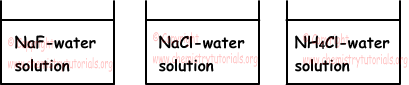EN | ES | DE | NL | RU

## Acids and Bases Exam2 and Problem Solutions

Acids and Bases Exam2 and  Problem Solutions

1. If we mix NaOH, HCl, HNO3 and KNO3 that all have equal molar concentration and volumes, which one of the following  ions has higher molar concentration?

I. H+

II. NO3-

III. Na+

IV. Cl-

V. K+

Solution:

We take 1 L from each solution, means we take 1 mole from each matter;

1mol NaOH, gives 1mol Na+ and 1mol OH- ions

1mol HCl, gives 1mol H+ and 1mol Cl- ions

1mol HNO3, gives 1mol H+ and 1mol NO3- ions

1mol KNO3, gives 1mol K+ and 1mol NO3- ions

In mixture following neutralization reaction occurs between ions;

H+(aq) + OH+ (aq) → H2O(l)

Since 1mol H+ and OH- is used, 1mol H+ stays. Thus, as you can see ion concentrations above, 2mol NO3- has the larger concentration value.

2. We mix 200 mL and 0,25M H2SO4 solution with 300 mL and 0,50M NaOH solution, find pH of this mixture?

Solution:

• Mole of H2SO4;

nH2SO4=V.M=(0,2).(0,25)=0,05mol

Since H2SO4 is strong acid it gives 2 x 0,05=0,1mol H+ ion to solution.

Mole of NaOH;

nNaOH=V.M=(0,3).(0,5)=0,15mol

Since NaOH is strong base it gives 0,15mol OH- ion to solution.

Neutralization reaction becomes;

H+ + OH- → H2O(l)

After reaction there are;

0,15 - 0,10 = 0,05mol OH- ion.

Volume of mixture;

V=0,2 + 0,3 =0,5 L

Molar concentration of OH- ion after reaction becomes;

[OH-]=nOH-/V=0,05/0,5=0,1=10-1M

Molar concentration of H+ ion after reaction becomes;

[H+]=(1 x 10-14)/[OH-]

[H+]=1 x 10-13M

pH=-log[H+]

pH=-log(10-13)

pH=13

3. Which ones of the following statements are true for 100 mL, 0,1 molar KOH solution?

I. It gives neutralization reaction with 0,1 molar 100 mL HCl solution

II. It gives neutralization reaction with 0,1 molar 100 mL HCN solution

III. If we mix it with 0,1molar 100 mL HCN, medium shows basic property

Solution:

Mole of KOH;

nKOH=V.M=(0,1).(0,1)=0,01mol

I. Mole of HCl;

nHCl=V.M=(0,1).(0,1)=0,01mol

Neutralization reaction;

KOH + HCl → KCl + H2O

0,01mol KOH reacts with 0,01mol HCl, I is true.

II. Mole of HCN;

nHCN=V.M=(0,1).(0,1)=0,01mol

Neutralization reaction;

KOH + HCN → KCN + H2O

0,01mol KOH reacts with 0,01mol HCN, II is true.

III. Since KCN is a strong base and weak acid salt, medium is basic. III is true.

4. Which ones of the following statements are falso for water solutions of strong bases?

I. They conduct electric current

II. Their H+ concentrations are larger than water

III. They react with acids and forms salt

Solution:

I. Strong bases conduct electric current, I is true.

II. In pure water; [H+]=[OH-]=1 x 10-7M, Adding base increases OH- concentration and decrease H+ concentration. II is false.

III. Strong bases react with acids and forms salt, III is true.

5. Which ones of the following solutions are neutral?NaOH and HCl are strong electrolytes and NH3 and HF are weak electrolytes.

Solution:

NaOH is strong base and HClis strong acid. NH3 is weak base and HF is weak acid.

I. In first solution there is salt formed by strong base and weak acid. Thus, NaF solution is basic.

II. In second solution NaCl is a salt formed by strong base and strong acid. So, NaCl solution is neutral

III. In third solution, NH4Cl is a salt formed by strong acid and weak base. So, solution is acidic.

The Original Author: